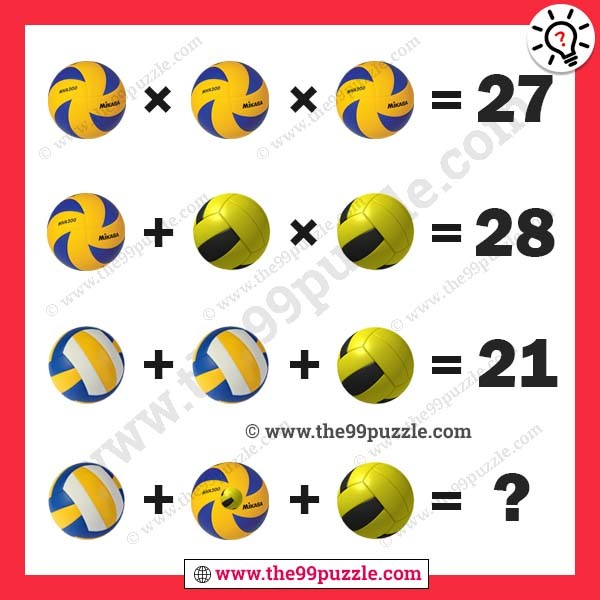# Ball picture math puzzle for genius – Puzz167

Ball picture math puzzle for genius. Math logic brain teaser for genius minds. These picture puzzles are challenging for people. Can you calculate the math equation picture puzzle?3×3×3=27

3+5×5=28

8+8+5=21

8+(3+5)+5=21